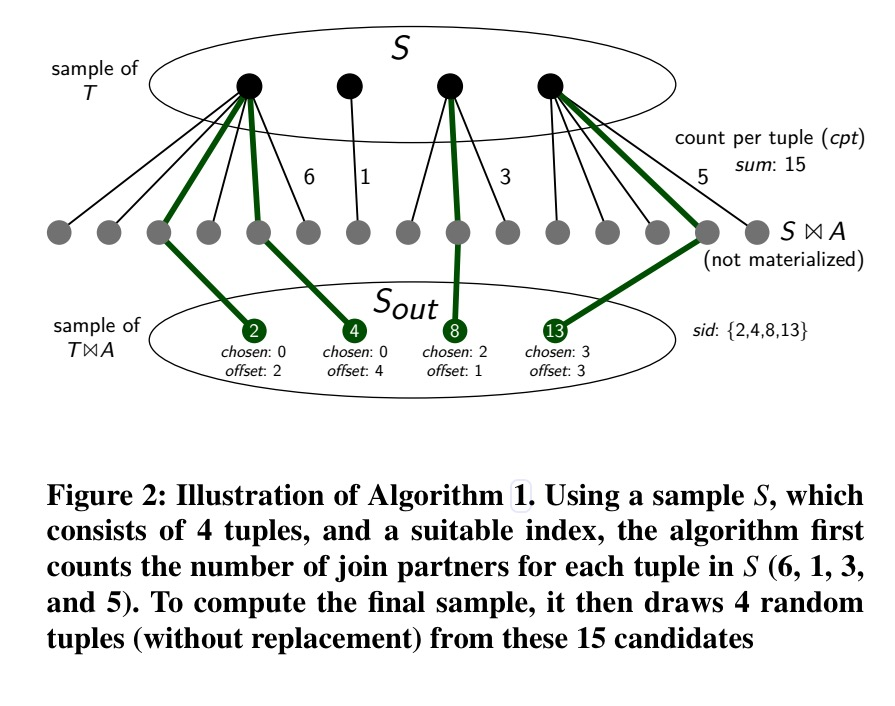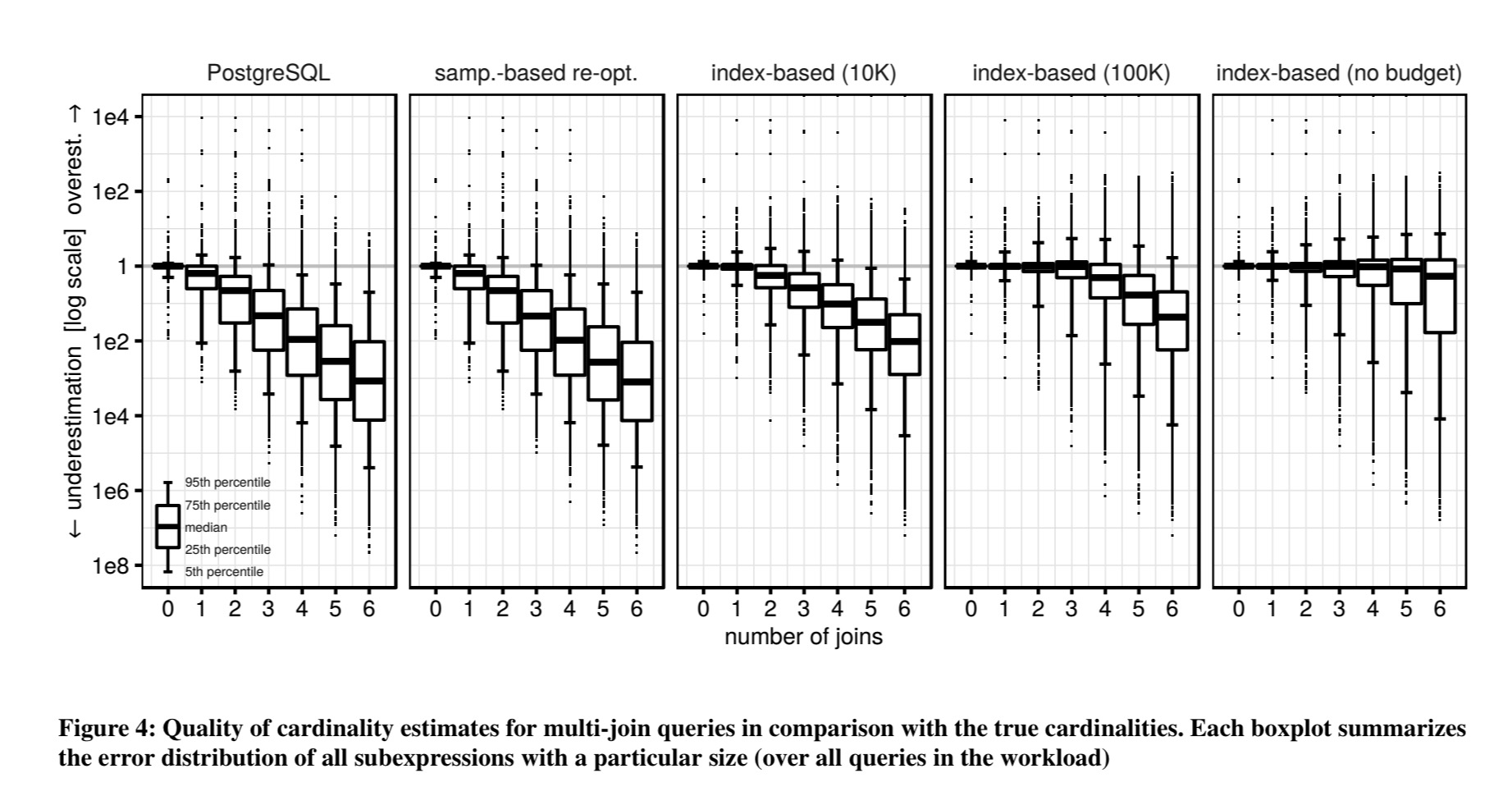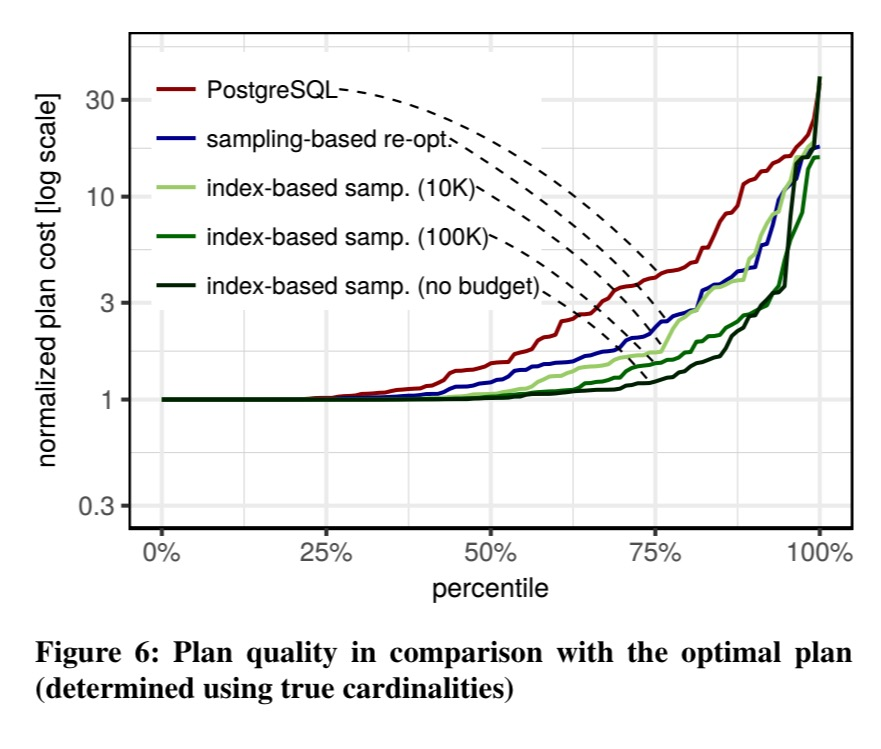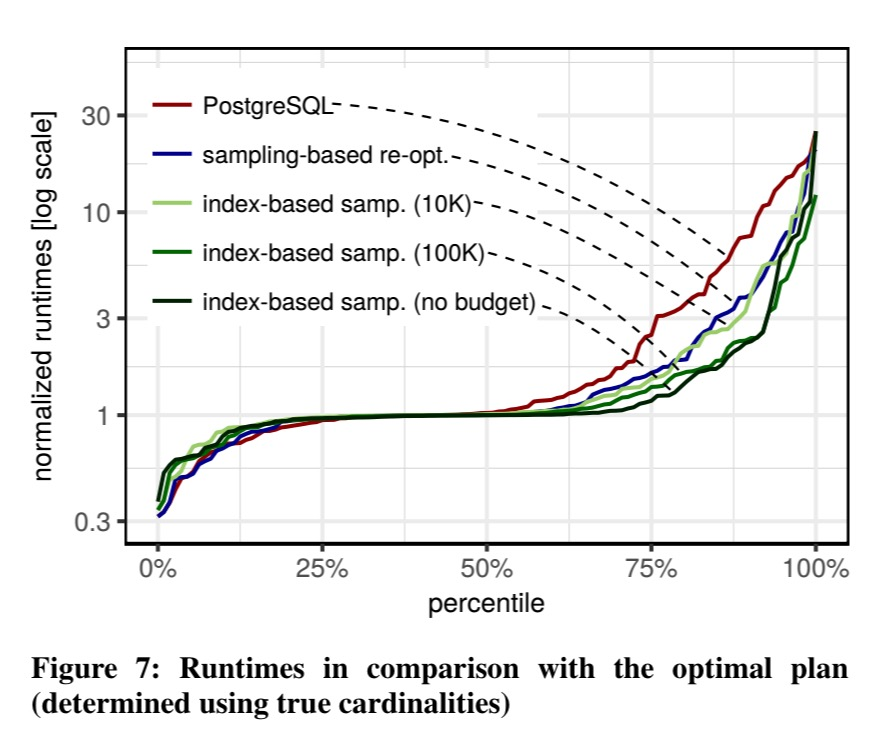# Cardinality estimation done right: index-based join sampling

## Cardinality estimation done right: Index-based join sampling

Cardinality estimation done right: Index-based join sampling Leis et al., CIDR 2017

Let’s finish up our brief look at CIDR 2017 with something closer to the core of database systems research – query optimisation. For good background on this topic a great place to start is Selinger’s 1979 classic ‘Access path selection in a relational database management system‘ (System R). One of the trickiest areas in query optimisation is determining the best join strategy, and in the almost 40 years since that System R paper, a lot of work has been done on this problem. If you are joining n tables, the number of possible join orders grows with n! .

When estimating the potential costs of different join orders, a fundamental input is an estimation of the number of matching tuples in each of the relations to be joined (aka cardinality estimation).

Virtually all industrial-strength systems estimate cardinalities by combining some fixed-size, per-attribute summary statistics (histograms) with strong assumptions (uniformity, independency, inclusion, ad hoc constants). In other words, most databases try to approximate an arbitrarily large database in a constant amount of space… For real-world data sets, cardinality estimation errors are large and occur frequently. These errors lead to slow queries and unpredictable performance.

Instead of using histograms to try and guess, for example, how many tuples a relation might have where$x=1$ an alternative approach would be to sample a subset of the rows and see how many matches there are in the sampled subset. Sampling has not traditionally been used for two reasons:

1. The disk I/O involved in going and fetching sample tuples is too slow, compounded by…
2. You need to sample a surprisingly large number of tuples in order to ensure that you’re still left with enough tuples to carry on estimating after joining. Say we’re joining A, B, and C. We want to estimate the cost of joining A and B, and then joining the result with C. We need enough samples remaining after the first join to be able to do a good enough estimation of the join with C. It turns out that with random sampling without replacement, in order to have n expected result tuples after the first join, we need to sample on the order of$\sqrt{n|A|}$ tuples. The more joins we have, the larger the initial samples need to be….

For in-memory databases, the first consideration largely goes away – we can sample reasonable numbers of tuples in a small amount of time (though presumably we always still have some kind of space budget we also have to adhere to). To address the second consideration it would be ideal if the samples we took were somehow more likely to ‘survive’ the joining process, so that a sufficient number of tuples flow through to the next join…

In this work we propose a novel cardinality estimation technique that produces accurate results but is much cheaper than joining random samples. The basic building block is an efficient sampling operator that utilizes existing index structures: to get an estimate for$A \Join_{A.p=B.f} B$, we obtain a random sample of$A$ and then look up the samples’ join partners in the index for$B.f$ (we could also start with$B$ using an index on$A.p$). The resulting sample for$A \Join B$ can be used as a starting point for obtaining a sample for$A \Join B \Join C$ by using an index on the join attribute$C.g$ and so on.

Suppose we have a sample S as a result of sampling some relation T, and for the next step we want to create a sample of$T \Join A$. For each tuple in S we use the index to look up how many matching tuples are expected in A. Summing these gives us the total expected number of matching tuples, and the required number of samples is then drawn from this set.The index-based sampling operator can cheaply compute a sample for a join result, but it is not a full solution by itself. We also need a join enumeration strategy which can systematically explore the intermediate results of a query using the sampling operator, while also ensuring that the overall sampling time is limited. If we sampled every possible combination, it would take too long for queries with many joins. In the Join Order Benchmark (JOB), queries with 7 joins have 84-107 intermediate results, and queries with 13 joins have 1,517-2,032. A time limit is set on the sampling phase, after which the algorithm falls back to traditional estimation.

The advantage is that one quickly obtains accurate estimates for large intermediate results. The disadvantage is that many small intermediate results are not sampled and thus have to be estimated using traditional estimation. It is well known that—due to the independence assumption—traditional estimators tend to underestimate result sizes. Therefore, when this mix of (accurate) sampling-based estimates and traditional (under-)estimates are injected into a query optimizer, it will often pick a plan based on the traditional estimates (as they appear to be very cheap). This phenomenon has been called “fleeing from knowledge to ignorance” and—paradoxically—causes additional, accurate information to decrease plan quality.

To address this issue, joins are sampled ‘bottom-up’ – i.e., first all 2-way joins are computed, then all 3-way joins, and so on. “A cost-based query optimizer will thus have precise knowledge for the costs of the early (and often crucial) joins.”

Integrating this approach into an existing DBMS is pretty straightforward since you just need to inject the results into the cardinality estimation component of the query optimizer, and no changes to the cost model or plan space enumeration algorithm are necessary.

Any query optimizer change that increases the performance for the vast majority of queries, will also decrease performance for some queries, which is very undesirable in production systems. Existing database systems are therefore very conservative with query optimizer changes. Thus, one could use our approach as an optional tuning feature for queries that are slower than expected. In other words, if a user is not satisfied with the performance of a particular query, to get better performance she may turn on index-based sampling only for that query.

### How well does it work?

Evaluation is based on the Join Order Benchmark, based on the Internet Movie Database. There are 113 queries with 3 to 16 joins. As a worst case for planning complexity, indices are created on all primary key and foreign key columns. To compare with traditional cardinality estimates, the test harness supports injection of cardinality estimates from an outside system – in this case obtained from PostgreSQL using the EXPLAIN command.

You can see that compared to the PostgreSQL estimates (column 1), index-based sampling with even just 10K samples produces much more accurate estimates. Also note how estimation accuracy decreases with the number of joins. The more samples we have of course, the closer we get to the true cardinalities.Do these more accurate cardinality estimates actually lead to better plans? In figure 6 below you can see the plan quality (log scale) of the plans produced. The cost of each query is normalized by the cost of the optimal plan that would have been chosen if the true cardinalities were known.Using PostgreSQL’s estimates, only around one quarter of the plans are close to the optimum, 42% of the plans are off by a factor of 2 or more, and 12% are off by a factor of 10 or more…

With a budget of 100,000 index lookups, index-based sampling improves performance for many queries – only 17% are off by a factor of 2 or more, and only 3% by a factor of 10 or more.

As expected, these better plans lead (mostly!) to faster runtimes:A small number of plans are actually faster (up to 3x) with inaccurate estimates rather than with the true cardinalities.

This effect is caused by cost model errors rather than inaccurate cardinalities and explains the hesitation of many commercial database systems to change their query optimizers.